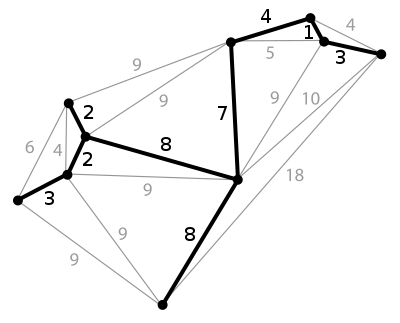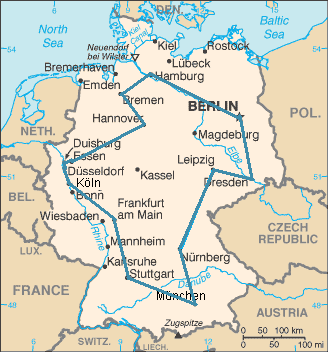# Combinatorial optimizationA minimum spanning tree of a weighted planar graph. Finding a minimum spanning tree is a common problem involving combinatorial optimization.

In applied mathematics and theoretical computer science, combinatorial optimization is a topic that consists of finding an optimal object from a finite set of objects. In many such problems, exhaustive search is not feasible. It operates on the domain of those optimization problems, in which the set of feasible solutions is discrete or can be reduced to discrete, and in which the goal is to find the best solution. Some common problems involving combinatorial optimization are the traveling salesman problem ("TSP") and the minimum spanning tree problem ("MST").

Combinatorial optimization is a subset of mathematical optimization that is related to operations research, algorithm theory, and computational complexity theory. It has important applications in several fields, including artificial intelligence, machine learning, mathematics, auction theory, and software engineering.

Some research literature considers discrete optimization to consist of integer programming together with combinatorial optimization (which in turn is composed of optimization problems dealing with graph structures) although all of these topics have closely intertwined research literature. It often involves determining the way to efficiently allocate resources used to find solutions to mathematical problems.

## Applications

Applications for combinatorial optimization include, but are not limited to:

• Developing the best airline network of spokes and destinations
• Deciding which taxis in a fleet to route to pick up fares
• Determining the optimal way to deliver packages
• Determining the right attributes of concept elements prior to concept testing

## Methods

There is a large amount of literature on polynomial-time algorithms for certain special classes of discrete optimization, a considerable amount of it unified by the theory of linear programming. Some examples of combinatorial optimization problems that fall into this framework are shortest paths and shortest path trees, flows and circulations, spanning trees, matching, and matroid problems.

For NP-complete discrete optimization problems, current research literature includes the following topics:

• polynomial-time exactly solvable special cases of the problem at hand (e.g. see fixed-parameter tractable)
• algorithms that perform well on "random" instances (e.g. for TSP)
• approximation algorithms that run in polynomial time and find a solution that is "close" to optimal
• solving real-world instances that arise in practice and do not necessarily exhibit the worst-case behavior inherent in NP-complete problems (e.g. TSP instances with tens of thousands of nodes).

Combinatorial optimization problems can be viewed as searching for the best element of some set of discrete items; therefore, in principle, any sort of search algorithm or metaheuristic can be used to solve them. However, generic search algorithms are not guaranteed to find an optimal solution, nor are they guaranteed to run quickly (in polynomial time). Since some discrete optimization problems are NP-complete, such as the traveling salesman problem, this is expected unless P=NP.

## Specific problemsAn optimal traveling salesperson tour through Germany’s 15 largest cities. It is the shortest among 43,589,145,600 possible tours visiting each city exactly once.

## Notes

1. Schrijver 2006, p. 1.
2. "Discrete Optimization". Elsevier. Retrieved 2009-06-08.
3. Take one city, and take all possible orders of the other 14 cities. Then divide by two because it does not matter in which direction in time they come after each other: 14!/2 = 43,589,145,600.

## References

• Das, Arnab; Chakrabarti, Bikas K, eds. (2005). Quantum Annealing and Related Optimization Methods. Lecture Notes in Physics. 679. Springer.
• Das, Arnab; Chakrabarti, Bikas K (2008). "Colloquium: Quantum annealing and analog quantum computation". Rev. Mod. Phys. 80: 1061. doi:10.1103/RevModPhys.80.1061.
• Lawler, Eugene (2001). Combinatorial Optimization: Networks and Matroids. Dover. ISBN 0-486-41453-1.
• Papadimitriou, Christos H.; Steiglitz, Kenneth (July 1998). Combinatorial Optimization : Algorithms and Complexity. Dover. ISBN 0-486-40258-4.
• Schrijver, Alexander (2003). Combinatorial Optimization: Polyhedra and Efficiency. Algorithms and Combinatorics. 24. Springer.
• Schrijver, Alexander (2005). "On the history of combinatorial optimization (till 1960)". In Aardal, K.; Nemhauser, G.L.; Weismantel, R. Handbook of Discrete Optimization (PDF). Elsevier. pp. 1–68.